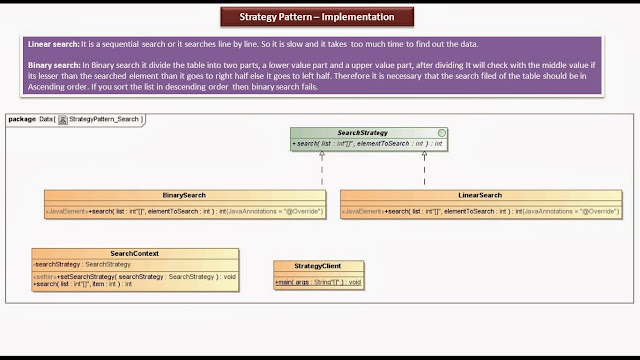## Monday, 30 December 2013

### Strategy Design pattern - Implementation [Search]

Click the below Image to EnlargeStrategy Design pattern - Implementation [Search]Strategy Design pattern - Implementation [Search] - Class Diagram

SearchStrategy.java

public interface SearchStrategy
{
int search(int[] list, int elementToSearch);
}

BinarySearch.java

public class BinarySearch implements SearchStrategy
{

@Override
public int search( int[] list, int elementToSearch )
{

int position = 0;

for (int i = 0; i < list.length; i++)
{
if (list[i] == elementToSearch)
{
position = i;
break;
}
}

System.out.println("Position of the element has been found using BinarySearch Algorithm");

return position;
}

}

LinearSearch.java

public class LinearSearch implements SearchStrategy
{

@Override
public int search( int[] list, int elementToSearch )
{
int beg = 0;
int end = list.length - 1;
int mid = (int) ((beg + end) / 2);
int position = 0;

while( beg <= end && list[mid] != elementToSearch )
{
if( elementToSearch < list[mid] )
end = mid - 1;
else
beg = mid + 1;

mid = (int) ((beg + end) / 2);
}

if( list[mid] == elementToSearch )
position = mid;
else
position = 0;

System.out.println("Position of the element has been found using LinearSearch Algorithm");

return position;
}

}

SearchContext.java

public class SearchContext
{

private SearchStrategy searchStrategy;

public void setSearchStrategy( SearchStrategy searchStrategy )
{
this.searchStrategy = searchStrategy;
}

public int search( int[] list, int item )
{
return searchStrategy.search(list, item);
}
}

StrategyClient.java

import java.util.Scanner;

public class StrategyClient
{
public static void main( String[] args )
{

System.out.println("Please enter Search Algorithm  : 'Linear' or 'Binary'");
Scanner scanner = new Scanner(System.in);
String searchAlgorithm = scanner.next();
System.out.println("Search Algorithm is : " + searchAlgorithm);

System.out.println("\nPlease enter element to be searched : ");
Scanner scanner1 = new Scanner(System.in);
int elementToSearch = scanner1.nextInt();
System.out.println("ElementToSearch is : " + elementToSearch);

SearchContext context = new SearchContext();

if( "Linear".equalsIgnoreCase(searchAlgorithm) )
{
context.setSearchStrategy(new LinearSearch());
}
else if( "Binary".equalsIgnoreCase(searchAlgorithm) )
{
context.setSearchStrategy(new BinarySearch());
}

int[] sortedList = { 1, 2, 3, 4, 5, 6, 7, 8 };

int position = context.search(sortedList,elementToSearch);

System.out.println("Position of the element is : "+ position);

}
}

Output

Please enter Search Algorithm  : 'Linear' or 'Binary'
Linear
Search Algorithm is : Linear

Please enter element to be searched :
5
ElementToSearch is : 5
Position of the element has been found using LinearSearch Algorithm
Position of the element is : 4

Please enter Search Algorithm  : 'Linear' or 'Binary'
Binary
Search Algorithm is : Binary

Please enter element to be searched :
7
ElementToSearch is : 7
Position of the element has been found using BinarySearch Algorithm
Position of the element is : 6

• Strategy Design pattern - Introduction
• Strategy Design pattern - Real Time Example [Compress files]
• Strategy Design pattern - Real Time Example [Payment]
• Strategy Design pattern - Real Time Example [Travel]
• Strategy Design pattern - Real Time Example [Sorting]
• Strategy Design pattern - Real Time Example [Search]
• Strategy Design pattern - Class Diagram
• Strategy Design pattern - Sequence Diagram
• Strategy Design pattern - Implementation [Compress files]
• Strategy Design pattern - Implementation [Travel]
• Strategy Design pattern - Implementation [Payment]
• Strategy Design pattern - Implementation [Sort]
• Strategy Design pattern - KeyPoints